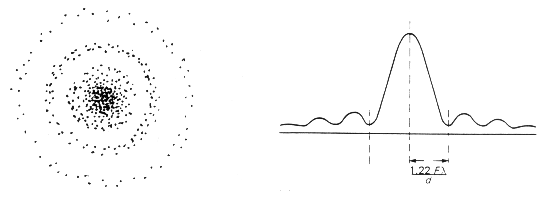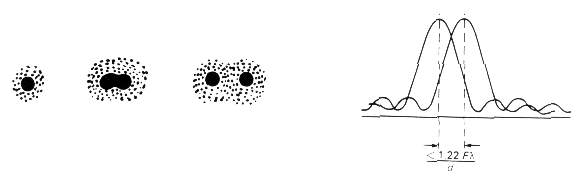BOT/MICRO/ZOOL 5364

Limits to Resolution in the Transmission Electron Microscope

It is desirable to understand several of the fundamental principles of light optics in order to understand the limitations of electron microscopy.

Diffraction. First, it is impossible to achieve absolute focus using any optical system that uses particles with wave-like properties, because of diffraction and interference. Diffraction results when a wavefront is impeded by any object, and of course the edge of the lens area constitutes an object, as does any superimposed aperture. Below is an example of how diffraction changes the wavefront in the presence of a small aperture. Notice that this causes the parallel wavefront to emerge from the aperture as a spherical wavefront.Airy disc. Second, using even a "perfect" optical system, a point of light cannot be focused as a perfect dot. Instead, the image when viewed critically consists of a disc composed of concentric circles with diminishing intensity. This is known as an Airy disc and is represented below.Resolution. Notice the primary, secondary and tertiary wavefronts generated by the Airy disc. (Of course, these continue to emanate at higher orders, but their affect on optical phenomena diminishes in importance with each higher order.) The resolution is typically described as the distance between the first order peak and the first order trough (designated "r" above). Resolution is empirically described as the ability to discriminate between two points. If an object is just below the level of resolution, the peaks generated by the two points will make the object appear to be a single point.Abbe's equation. Resolution in a perfect optical system can be described mathematically by Abbe's equation. In this equation:

d = _0.612 * l_
n sin a

where:

d = resolution
l
n
= index of refraction of medium between point source and lens, relative to free space
a
= half the angle of the cone of light from specimen plane accepted by the objective (half aperture angle in radians)
n
sin a is often expressed as NA (numerical aperture)

This is the diffraction-limited resolution of an optical system. If all aberrations and distortions are eliminated from the optical system, this will be the limit to resolution. If aberrations and distortions are present, they will determine the practical limit to resolution.

De Broglie equation. By combining some of the principles of classical physics with the quantum theory, de Broglie proposed that moving particles have wave-like properties and that their wavelength can be calculated, based on their mass and energy levels. The general form of the de Broglie equation is as follows:

l = __h__
m * v

where:

l = wavelength
h = Planck's constant (6.6 X 10-27)
m = mass of the particle (9.1 X 10-28)
v = velocity of the particle

When an electron passes through a potential difference (accelerating voltage field) V, its kinetic energy with be equal to the energy of the field, i.e. eV (energy in electron volts) = V (the accelerating voltage). As you may recall, e = mc2. By restating this for velocities below the speed of light and particles with true mass, the energy of an electron may be stated as follows:

eV = 1/2 mv2

where:

eV = energy in electron volts (e = 4.8 X 10-10)
m = mass of the particle
v = velocity of the particle

By using some assumptions about the velocity of the particle and its mass, it is possible to express either wavelength (l) or velocity (v) in terms of the accelerating voltage (V). By further substituting the values of h and m above, the equation for l reduces to the following:

l = _1.23 nm_
V1/2

One caveat is that as the velocity of the electron approaches the speed of light, Einstein's special equations of relativity need to be used for greater accuracy as the mass and momentum of electrons increases with velocity.

Equation for resolution in TEM: This value for l can then be substituted into Abbe's equation. Since angle a is usually very small, for example 10-2 radians (a likely figure for TEM), the value of a approaches that of sin a , so we replace it. Since n (refractive index) is essentially 1, we eliminate it, and we multiply 0.612 by 12.3 to obtain 0.753. Therefore, the equation reduces to the following:

d = __0.753__
a V1/2

where:

d = resolution in nm
a = half aperture angle
V = accelerating velocity

Now, solving for 100,000 volts, the result is 0.24 nm or 2.4 Å. This improves with higher accelerating voltage and gets worse with lower voltages. (Using Einsteinian calculations, the resolution is: 0.22 nm or 2.2 Å.) Each lens and aperture has its own set of aberrations and distortions. If aberrations and distortions are present, they will determine the practical limit to resolution.